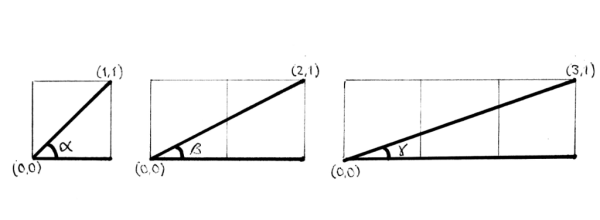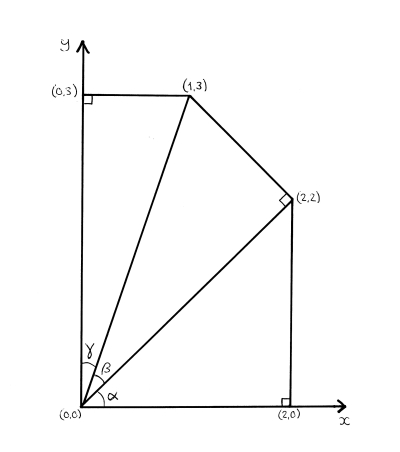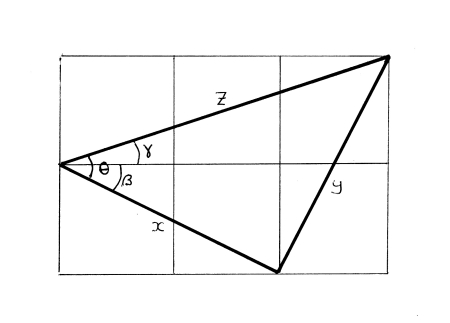# How Does the Imaginary Square Root of -1 Earn its Dinner?

by Jessica Collins

This is an attempt to recapture a feeling of queasiness I felt in my early teens, when I was already captivated by mathematics, but didn't yet know much about mathematics. It's an attempt to recapture that feeling of queasiness and then to resolve it in a way that it might have found resolution at the time, had I been asked the right questions.

Puzzle

I'm going to proceed somewhat indirectly and begin with a rather cute little geometrical problem. If you've got a few minutes to spare I encourage you to spend that time with pencil and paper trying to solve this puzzle before proceeding to read the rest of this piece, in which I'll describe three different ways of solving it. Here is the problem:

What is the sum of the three angles that the x-axis makes with the lines joining the origin to the points (1,1), (2,1), and (3,1) respectively?

The three angles are those shown in the following diagrams:The first angle α is obviously 45°. If you know some trigonometry you'll recall that given a right triangle one of whose other angles is θ, the tangent of the angle θ, tan(θ) for short, is the ratio of the length of the side of the triangle opposite the angle θ to the length of the side adjacent to the angle. Thus we can see from the diagram above that β, the angle that the line joining the origin to the point (2,1) makes with the x-axis, is the angle whose tangent is 1/2, and that the angle γ is the angle whose tangent is 1/3.

This means you could use the arctan or inverse tan (tan-1) function of a scientific calculator to find the answer to the question above. The question was asking in effect:

What is: tan-1(1) + tan-1(1/2) + tan-1(1/3) ?

If you do this on a calculator, you'll find that the three values are:

α = 45°
β = 26.565051177077989 . . . °
γ = 18.434948822922011 . . . °

and that these three angles sum exactly to 90°, which was not immediately obvious, at least for me, from looking at the diagram.

But, without recourse to the calculator, can you explain why this is so? Can you find a simple proof that α + β + γ = 90°? We may restate the problem:

Show that the three angles the x-axis make with the points (1,1), (1,2), and (1,3) respectively sum to a right angle.

This is the point at which you might pause for a few minutes with pencil and paper before proceeding to read what follows.

Solution

Just over a week ago I posed that geometrical problem on Facebook. Almost immediately my friend Kenny Easwaran responded with the following clever geometrical proof (I'm paraphrasing Kenny's words here):

First expand the triangle with corners at (0,0), (1,0), and (1,1) into the similar triangle (0,0), (2,0), (2,2). This expansion doesn't change the size of the angle α. (See diagram below.)

Now take the right triangle containing the angle β, i.e. the triangle whose corners are at (0,0), (2,0), and (2,1) and expand it, while rotating it about the origin, so that its base comes to coincide with the hypotenuse of that first expanded triangle. This second expanded and rotated triangle now has vertices at (0,0), (2,2), and (1,3). To see this, note that the latter triangle is indeed similar to its unexpanded, unrotated version, since it is still a right triangle one of whose legs is twice the length of the other. So the second expansion and rotation doesn't affect the size of angle β.

But now the angle between the y-axis and the hypotenuse of the second expanded, rotated triangle, i.e. the line connecting the origin to the point (1,3), must be γ, since the triangle (0,0), (0,3), (1,3) is the reflection of the triangle (0,0), (3,0), (3,1) through the line that bisects the x– and y-axes. Hence from this construction we can see that α + β + γ = 90°. QED.Shortly after Kenny posted his answer, I received a second and rather different proof from S. Abbas Raza, a proof based on the Theorem of Pythagoras and its converse. Pythagoras's Theorem states that for any right triangle, the square of the length of its hypotenuse is the sum of the squares of the lengths of its other two sides. The Converse Pythagorean Theorem states that if the square of the length of one side of a triangle is the sum of the squares of the lengths of the other two sides, then the triangle is a right triangle.

Abbas's proof refers to the following diagram. Again, I am paraphrasing his words:Consider the grid of six unit squares above. The angle θ = β + γ. We want to show that θ = 45°. By the Pythagorean Theorem:

x = √ (12 + 22) = √ 5
y = √ (12 + 22) = √ 5
z = √ (12 + 32) = √ 10

But now the triangle with sides x, y, and z has one side, z, whose length squared is the sum of the squares of the lengths of the other two sides. Hence by the Converse Pythagorean Theorem it must be a right triangle. But then it is a right triangle whose legs are of equal length, and so the angle θ = 45°. QED.

Now both these proofs are ingenious, and yet there is something ad hoc about each of them, in that each method requires you to hit upon the particular construction that allows the result to fall out in your lap. In the case of Kenny's method, you need to find the right ways of expanding, rotating, and reflecting the original triangles so that they fit the right angle separating the x– and y-axes. In the case of Abbas's proof, you must happen to hit upon the particular construction involving the six unit squares. It would be nice to have a more routine, systematic way of attacking a problem like this.

So now to the autobiographical part of my story.

Worry

My father is a mathematical physicist. I remember that, when I was thirteen or fourteen years old, he introduced me to the complex numbers. A complex number is a number of the form x + iy, where x and y are real numbers, and i is the square root of minus one.

As a kid, your immediate reaction to this is likely to be: Wait! There is no square root of minus one! But I quickly saw that that was question-begging. Of course there is no real number that is the square root of minus one, but that's like objecting to negative numbers by saying: Wait! There is no number that added to one yields zero! Of course there's no positive number that added to one yields zero. But so what? That's the whole point of introducing the negative numbers.

Even a mathematician as great as Leibniz, described the number i as that amphibian between existence and non-existence. Such a knee-jerk prejudice against complex numbers is no doubt reflected in and fostered by the unfortunate terminology that would have x be the real and iy the merely imaginary part of x + y. Of course the terminology that opposes positive numbers to negative is also pejorative to the latter, but it is only in the literal sense that negative numbers are lesser than.

So I thought this was all kind of cool, but I was skeptical about the point of any of it. I wasn't so much worried about the ontological issue, I was happy to allow that the existence of complex numbers was no more problematic than that of the negative numbers. But I couldn't see what they could possibly be good for? How do they earn their dinner? It all seemed to me a bit like an empty formal game. I didn't have this worry about negative numbers, because I could see they had a purpose: e.g. in talking about gains and losses. But complex numbers seemed suspect to me.

I pushed my father on this point.

Now being a physicist, he immediately started answering me by talking about electrical engineering and the convenience of representing an alternating current by a complex number which encodes both the amplitude and the phase of the current. Hmmm, that was not the kind of answer I wanted. I didn't know any of the theory of electricity at the time and in fact had little interest in physics, to my dad's disappointment. My eyes quickly glazed over. It would have done no more good to have been told that complex numbers are an indispensible tool in the formulation of quantum mechanics. At age thirteen I knew no quantum mechanics.

Resolution

I wish in retrospect that my father had answered my question by posing the earlier geometrical problem to me. That would have helped me enormously.

Because that geometrical problem has the following neat solution.

Suppose that the problem is posed on the complex plane. The complex number x + iy is represented on the complex (or Argand) plane as the point with coordinates (x, y). The x-axis is known as the real axis and the y-axis is the imaginary axis. Alternatively, you can represent x + iy as the pair (r, θ) where the modulus r is the distance of the point (x, y) from the origin, this is the amplitude in the complex number representation of an alternating electrical current, and θ, the argument, is the angle that the ray from the origin to (x, y) makes with the x-axis (by convention: going counterclockwise). This angle θ is the phase of the current.

So now we have an angle associated with each complex number, and these angles may be summed by multiplying the corresponding numbers. This means that the sum of the three angles in our geometrical problem is the angle associated with the complex number that is the product of the numbers represented by the points (1,1), (2,1), and (3,1).

This product is routinely obtained by simply multiplying out the expression:

(1 + i)*(2 + i)*(3 + i) = (2 + i + 2i -1)*(3 + i) = (1 + 3i)*(3 + i) = 3 + i + 9i – 3 = 10i

But that number, ten times the square root of minus one, corresponds to the point (0,10) which lies on the imaginary axis of the complex plane, that is, its argument or phase is 90 degrees, the angle separating the x-axis from the y-axis. QED.

Seeing this solution to the geometrical problem would have been enormously helpful in quelling the skeptical worries of my early teenage self about the usefulness of the complex numbers. The algebraic manipulation of complex numbers is not just the making of moves in an empty formal game. Imaginary numbers are shown here to be of help in straightforwardly solving a very real and down-to-earth geometrical problem. That's exactly the kind of justification for their introduction into mathematical practice that I had been seeking from my father: a justification that is itself entirely internal to mathematical practice, rather than one which reaches outside mathematics by appealing to applications to physics, for example.

When I posted this solution for my Facebook friends, Kenny Easwaran's response was Of course! As he pointed out to me, this method is actually not so different from his construction after all, because multiplication by a complex number corresponds to an expansion and a rotation in the complex plane. In particular, multiplication by the complex number (r, θ) amounts to an expansion by the factor r accompanied by a rotation counterclockwise around the origin through angle θ.

History

In fact the kind of internal mathematical justification of the complex numbers that I've been concerned with here, already figures in the history of mathematics at a number of places. Let me conclude with a brief mention of three such examples, which, however, would not have been as accessible to my thirteen year-old self as our geometric puzzle above.

(1) Girolamo Cardano, in his Ars Magna of 1545, noted that any cubic equation ax3 + bx2 + cx + d = 0 can be reduced to the form x3 = 3px + 2q and then showed that this equation has a solution that can be written as an explicit formula that involves the term q2 – p3. Twenty-seven years later Rafael Bombelli noticed that if p3 > q2 then this formula will involve complex numbers. Consider the equation x3 = 15x + 4. You can check that this has the solution x = 4. But here Cardano's explicit formula tells us that x is the sum of the cube roots of 2+11i and 2-11i. Bombelli used the assumption that Cardano's formula remains accurate in this case to reverse engineer the rules for adding and multiplying complex numbers. And he took the success of that to constitute evidence of the fruitfulness of complex arithemetic.

(2) The Fundamental Theorem of Algebra (d'Alembert 1746, Gauss 1799) states that every polynomial with complex coefficients has at least one complex root. An equivalent way of stating this is to say that the field of complex numbers is algebraically closed. This beautiful property also serves to illustrate the naturalness of the complex numbers as an object of mathematical investigation. The field of real numbers, by contrast, is not algebraically closed.

(3) The mathematician Felix Klein (1849-1925) is best known for his statement of the 1872 Erlangen Program that sought to characterize geometries by their underlying symmetry groups. Euclidean plane geometry, for example, is simply the study of those properties invariant under the group of similarities, where a similarity is a mapping of the plane onto itself that preserves ratios of distances. Let's say that a direct similarity is one which preserves left- and right-handedness. Then every direct similarity can be achieved by a translation (i.e. a sliding in a straight line across the plane) and a dilitative rotation (i.e. the kind of combination of a rescaling plus a rotation that we examined above). Now any translation is given by addition of a complex number and, as we noted before, any dilitative rotation is given by multiplication by a complex number. This means that by interpreting the Euclidean plane as complex plane, we see that the Euclidean plane, in a sense, provides its own representation in Erlangen Program terms. The Euclidean plane is very special indeed in this regard. The same is not even true for Euclidean three-space.

Notes

The first and the third examples from the section History are discussed in more detail in Tristan Needham Visual Complex Analysis (1997) Clarendon, Oxford, which is also the place from which I've drawn the quotation from Leibniz.

* * *

Jessica Collins (aka John Collins) is Associate Professor of Philosophy at Columbia University.